Ampere's circuital law|Magnetic effect of current

(4) Ampere's circuital law

• Ampere's circuital law in magnetism is analogous to gauss's law in electrostatics
• This law is also used to calculate the magnetic field due to any given current distribution
• This law states that
" The line integral of resultant magnetic field along a closed plane curve is equal to μ0 time the total current crossing the area bounded by the closed curve provided the electric field inside the loop remains constant" Thus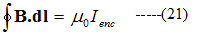where μ0 is the permeability of free space and Ienc is the net current enclosed by the loop as shown below in the figure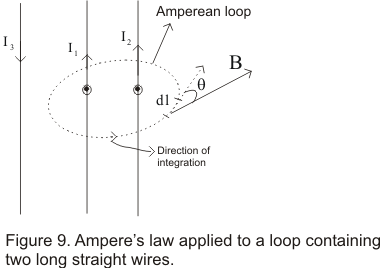• The circular sign in equation (21) means that scalar product B.dl is to be integrated around the closed loop known as Amperian loop whose beginning and end point are same
• Anticlockwise direction of integration as chosen in figure 9 is an arbitrary one we can also use clockwise direction of integration for our calculation depending on our convenience
• To apply the ampere's law we divide the loop into infinitesimal segments dl and for each segment, we then calculate the scalar product of B and dl
• B in general varies from point to point so we must use B at each location of dl
• Amperion Loop is usually an imaginary loop or curve ,which is constructed to permit the application of ampere's law to a specific situation

Proof Of Ampere's Law
• Consider a long straight conductor carrying current I perpendicular to the page in upward direction as shown below in the figure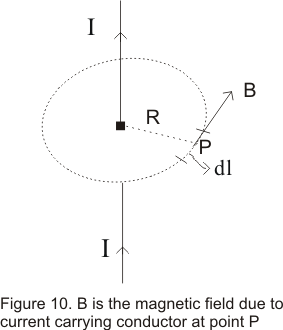• From Biot Savart law, the magnetic field at any point P which is at a distance R from the conductor is given by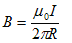• Direction of magnetic Field at point P is along the tangent to the circle of radius R withTh conductor at the center of the circle
• For every point on the circle magnetic field has same magnitude as given byAnd field is tangent to the circle at each point
• The line integral of B around the circle is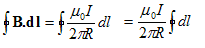since ∫dl=2πR ie, circumference of the circle so,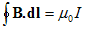This is the same result as stated by Ampere law
• This ampere's law is true for any assembly of currents and for any closed curve though we have proved the result using a circular Amperean loop
• If the wire lies outside the amperion loop, the line integral of the field of that wire will be zero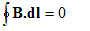but does not necessarily mean that B=0 everywhere along the path ,but only that no current is linked by the path
• while choosing the path for integration ,we must keep in mind that point at which field is to be determined must lie on the path and the path must have enough symmetry so that the integral can be evaluated

Note to our visitors :-

Thanks for visiting our website.
DISCLOSURE: THIS PAGE MAY CONTAIN AFFILIATE LINKS, MEANING I GET A COMMISSION IF YOU DECIDE TO MAKE A PURCHASE THROUGH MY LINKS, AT NO COST TO YOU. PLEASE READ MY DISCLOSURE FOR MORE INFO.